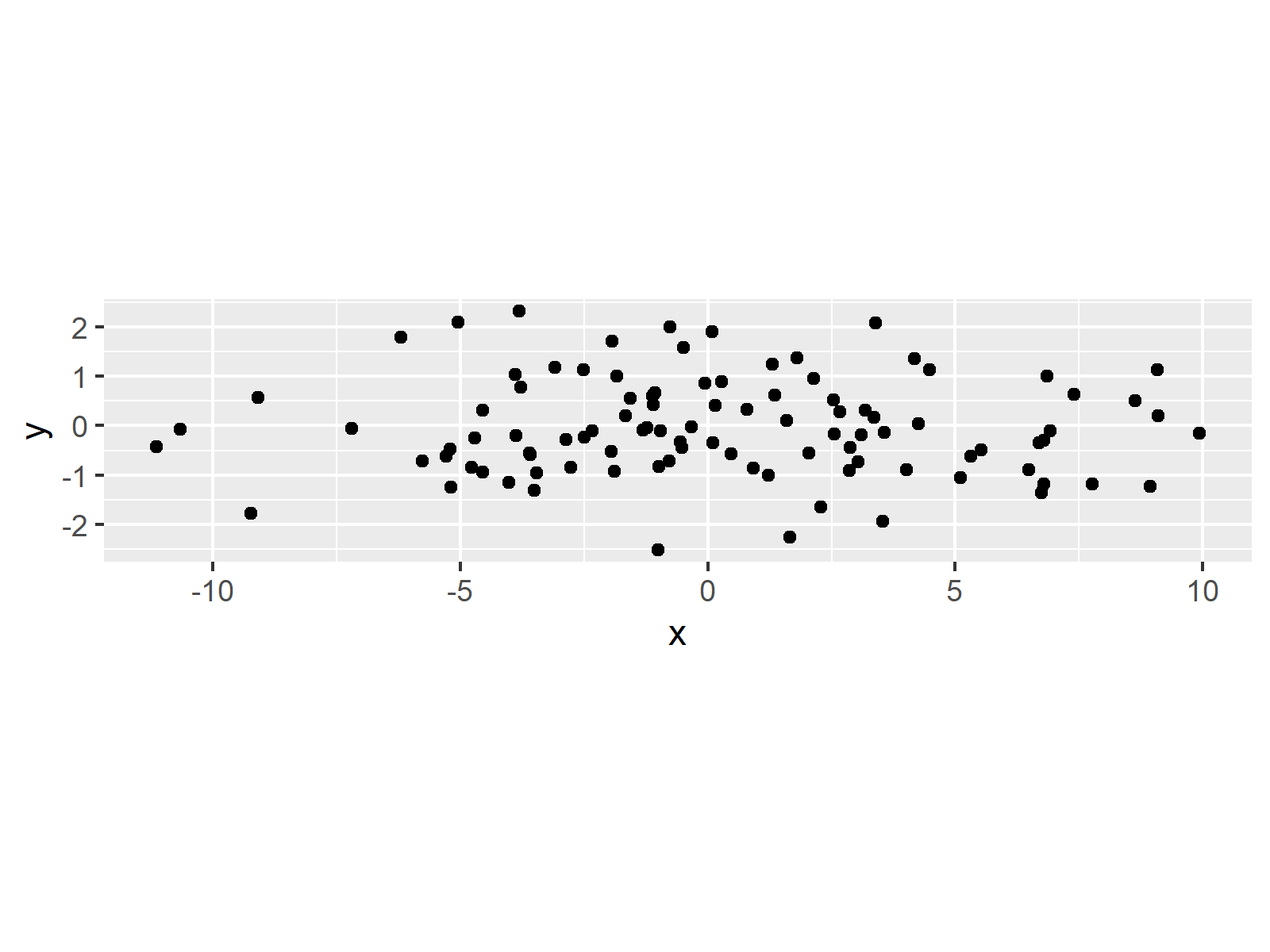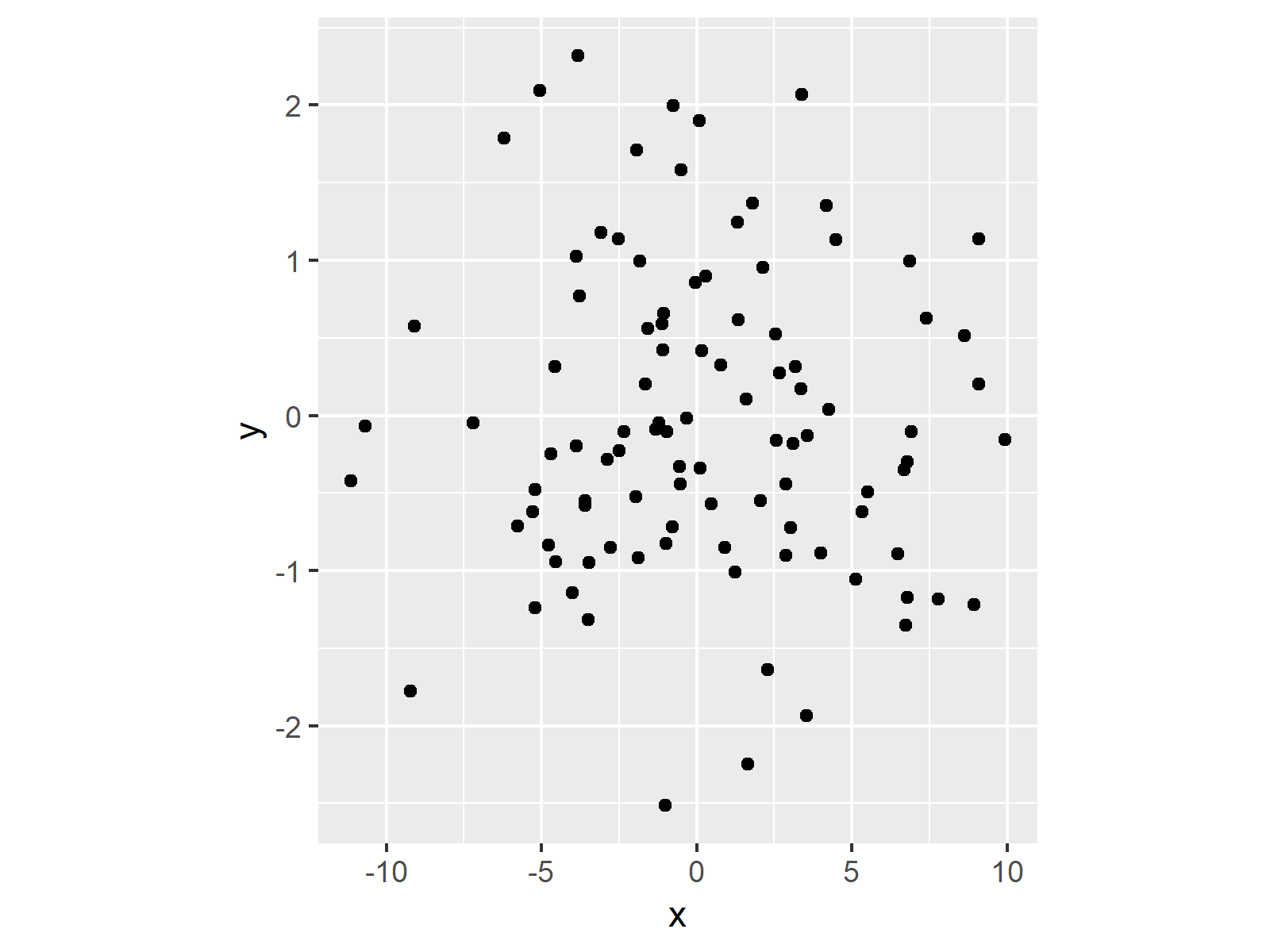# Fix Aspect Ratio in ggplot2 Plot in R (2 Examples)

In this R tutorial you’ll learn how to use the coord_fixed function to set a fixed aspect ratio.

The table of content is structured as follows:

Let’s jump right to the examples:

## Exemplifying Data, Add-On Packages & Default Plot

As a first step, we’ll need to create some example data:

```set.seed(347865)                    # Example data
data <- data.frame(x = rnorm(100, 0, 5),
y = rnorm(100))
#            x           y
# 1   5.320164 -0.62260359
# 2 -10.665791 -0.06837196
# 3  -3.872701 -0.19777942
# 4  -4.781276 -0.83737958
# 5  -4.704341 -0.24583468
# 6   2.868131 -0.44292606```

The previous output of the RStudio console shows that our exemplifying data has two numeric columns with the names x and y.

If we want to plot our data with the ggplot2 add-on package, we also need to install and load ggplot2:

```install.packages("ggplot2")         # Install & load ggplot2
library("ggplot2")```

Next, we can plot our data:

```ggp <- ggplot(data, aes(x, y)) +    # Basic ggplot2 plot
geom_point()
ggp                                 # Print basic ggplot2 plot```As shown in Figure 1, we drew a basic ggplot2 scatterplot with the previous R syntax.

## Example 1: Fixed Aspect Ratio Using coord_fixed Function

In this Example, I’ll illustrate how to set a fixed aspect ratio in a ggplot2 plot in R. Have a look at the following R code:

`ggp + coord_fixed()                 # Apply coord_fixed`The output of the previous R programming syntax is visualized in Figure 2: You can see a ggplot2 scatterplot with fixed proportions of the x- and y-axes. This fixed aspect ratio is set to 1 in this example.

## Example 2: Fixed Aspect Ratio Using coord_fixed Function & ratio Argument

Example 2 shows how to set aspect ratios with a different ratio than 1. In the example, we’ll use an aspect ratio of 5:

`ggp + coord_fixed(ratio = 5)        # Apply coord_fixed & ratio`The output of the previous R syntax is illustrated in Figure 3: As you can see the aspect ratio is still fixed, but with a different ratio of the two axes.

## Video, Further Resources & Summary

Would you like to know more about the ggplot2 package? Then you may have a look at the following video of my YouTube channel. I explain how to use the ggplot2 package in much more detail:

Please accept YouTube cookies to play this video. By accepting you will be accessing content from YouTube, a service provided by an external third party.If you accept this notice, your choice will be saved and the page will refresh.

In addition, you could have a look at the other tutorials of https://www.statisticsglobe.com/. You can find a selection of articles about the ggplot2 package below:

To summarize: In this post, I illustrated how to fix aspect ratios of ggplot2 graphics and change the proportions from square to rectangle in R programming. Don’t hesitate to let me know in the comments below, in case you have any further questions.

Subscribe to the Statistics Globe Newsletter

•August 3, 2023 7:23 pm

I believe the figures rendered with coord_fixed() and coord_fixed(5) are reversed.

•August 8, 2023 9:44 am